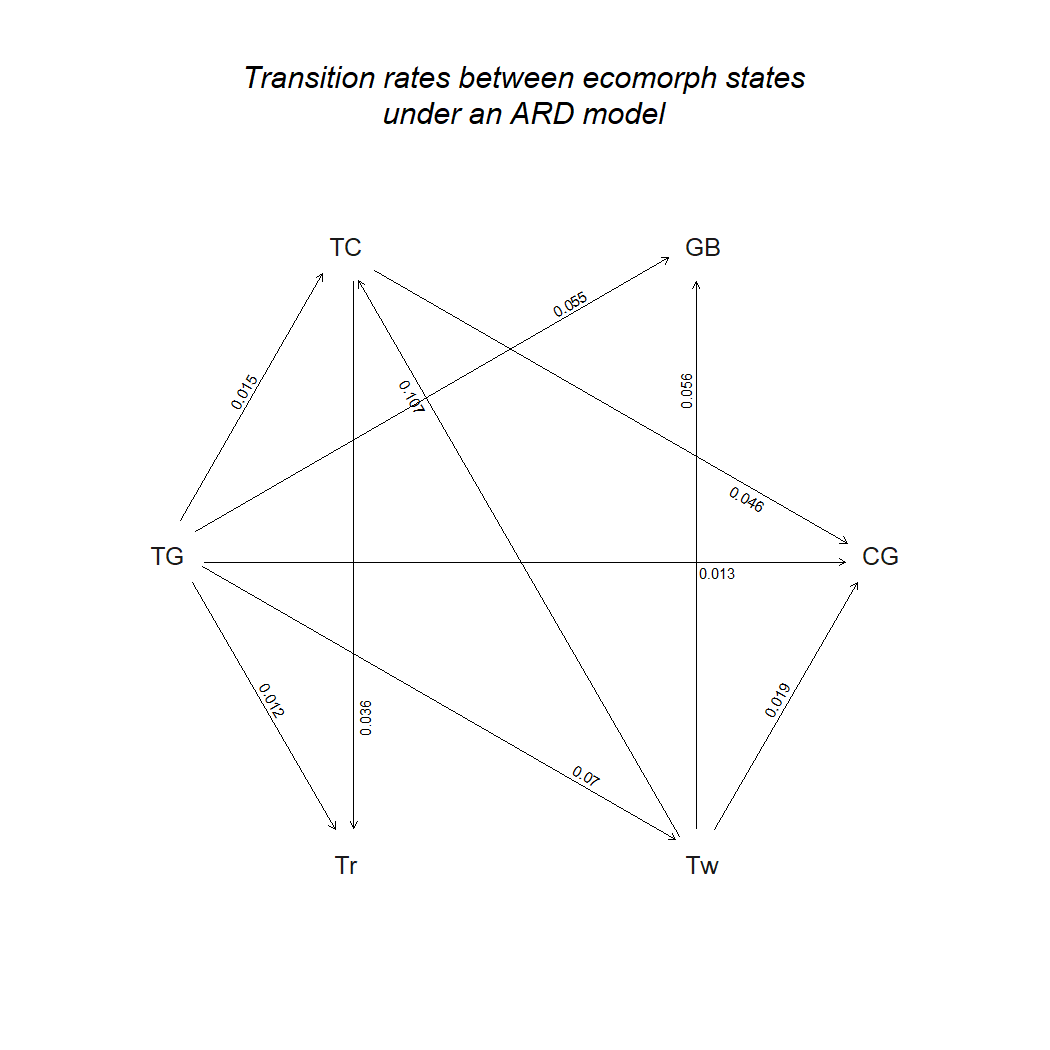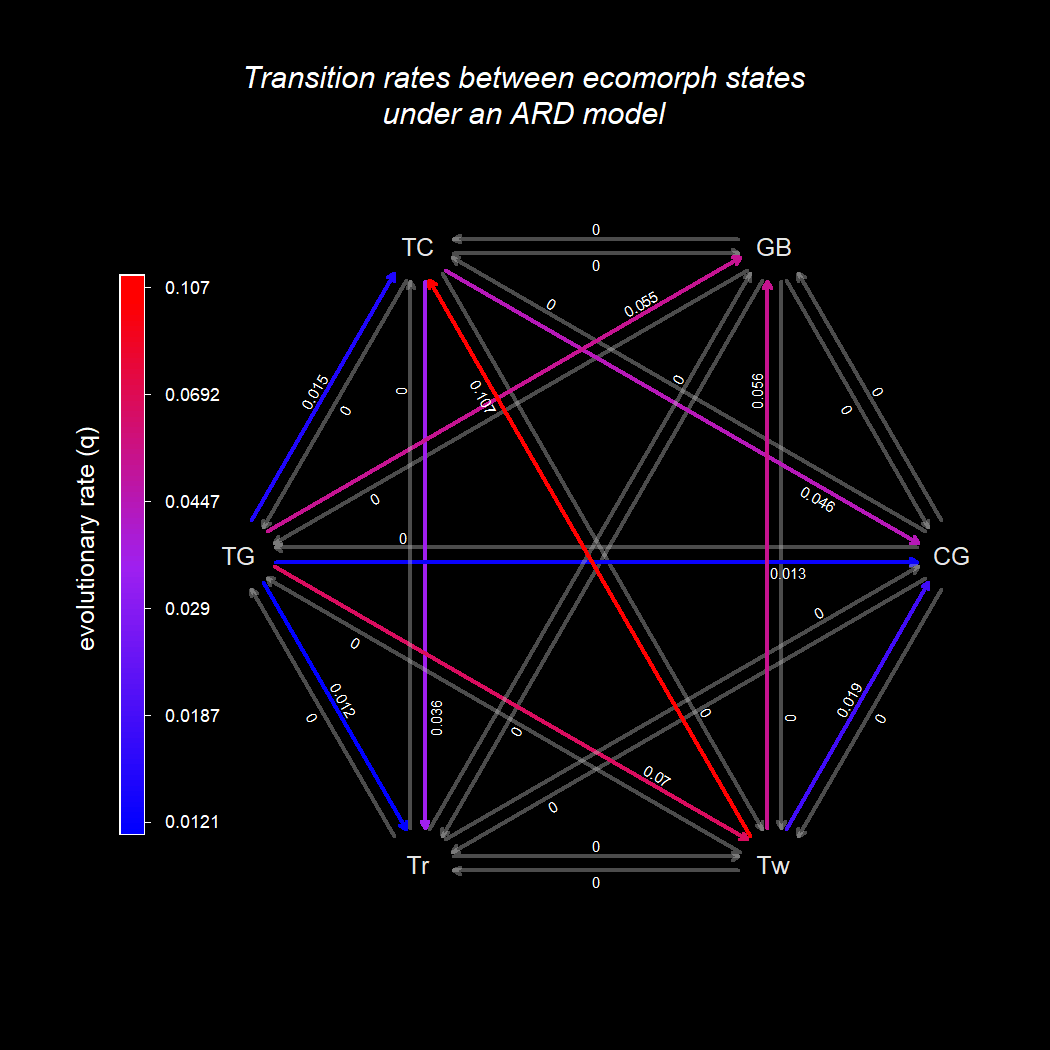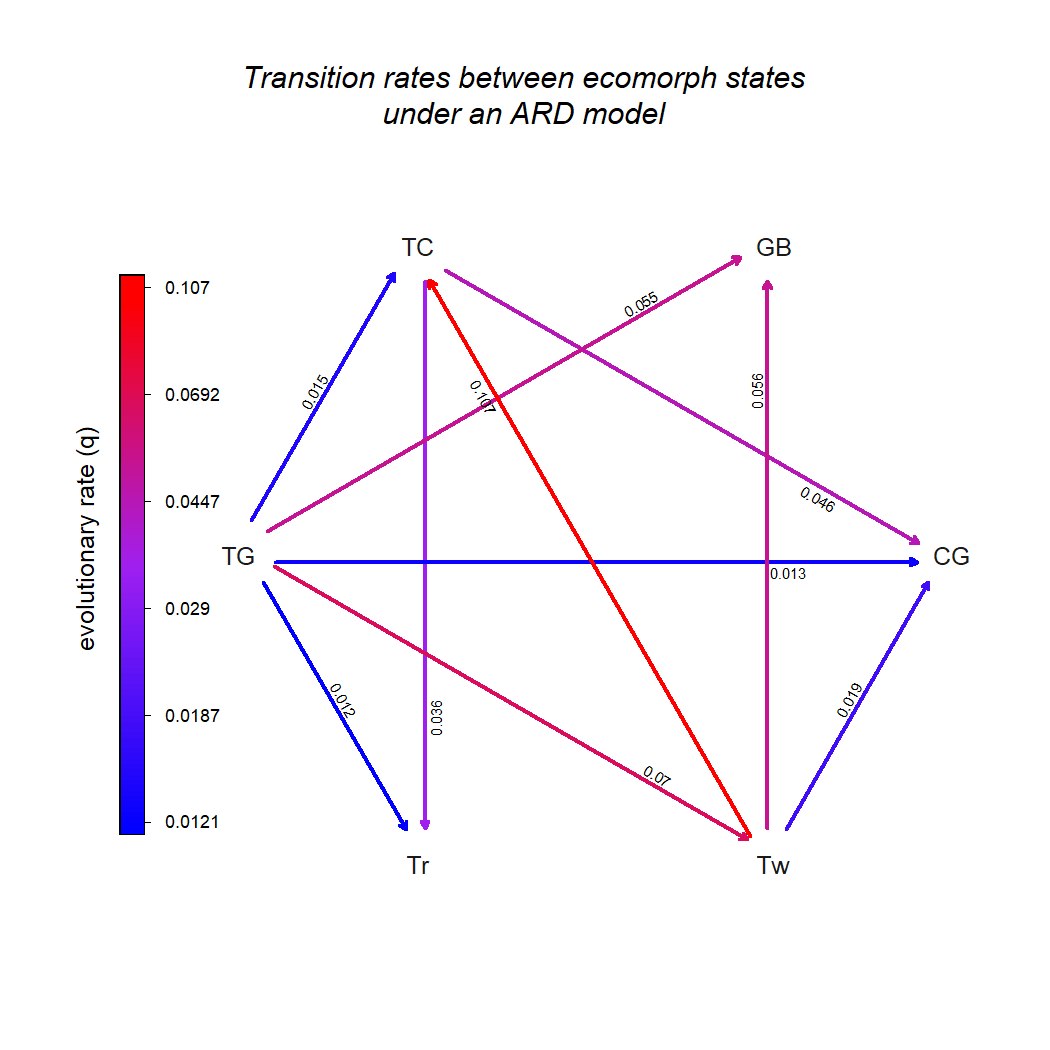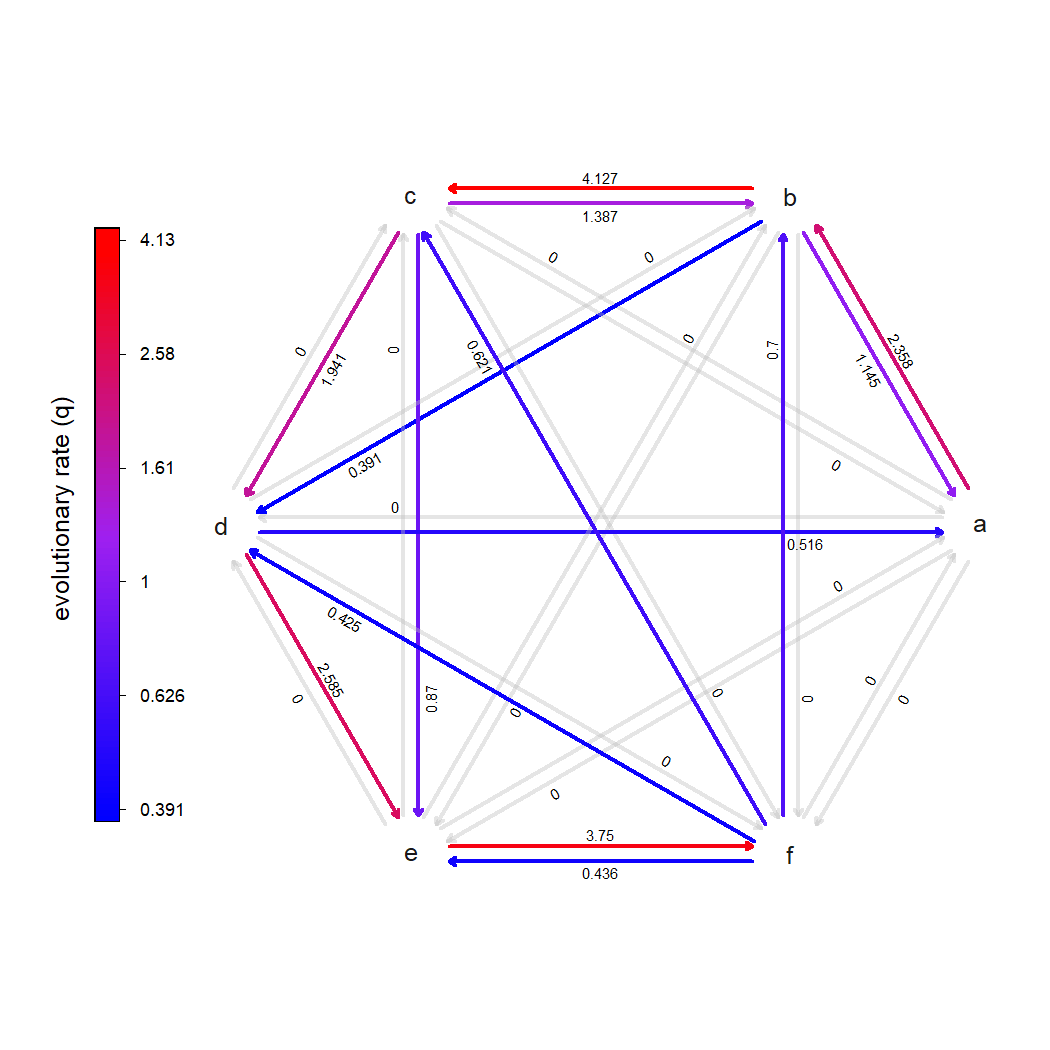## Thursday, June 17, 2021

### Update to plotting method for the rates among states under a fitted Mk model using phytools

I just pushed an update go the `plot.Qmatrix` S3 method in phytools (which is also used to plot `"fitMk"` and the `geiger::fitDiscrete` `"gfit"` object class) to visualize the different transition rates between discrete character states in a fitted Mk model using a “cool” to “hot” color gradient.

Here's a quick demo of how it works.

For starters, I'll load phytools which I've updated from it's GitHub page using devtools.

``````library(phytools)
packageVersion("phytools")
``````
``````##  '0.7.82'
``````

Simply for convenience, I'll use the tree of Anolis lizards and ecomorphological habitat specialization, as follows.

``````data(anoletree) ## load dataset & trait
ecomorph<-getStates(anoletree,"tips")
anoletree<-as.phylo(anoletree)
``````

Now we can go ahead and fit our model.

Because it'll create the most interesting visualization, I'll jump straight to the `"ARD"` “all-rates-different” model, even though this plotting method should work for any model of evolution.

``````fitARD<-fitMk(anoletree,ecomorph,model="ARD",
pi="fitzjohn")
fitARD
``````
``````## Object of class "fitMk".
##
## Fitted (or set) value of Q:
##          CG       GB        TC        TG       Tr        Tw
## CG 0.000000 0.000000  0.000000  0.000000 0.000000  0.000000
## GB 0.000000 0.000000  0.000000  0.000000 0.000000  0.000000
## TC 0.046320 0.000000 -0.082524  0.000000 0.036204  0.000000
## TG 0.013046 0.055357  0.015068 -0.165306 0.012121  0.069713
## Tr 0.000000 0.000000  0.000000  0.000000 0.000000  0.000000
## Tw 0.018787 0.055802  0.106892  0.000000 0.000000 -0.181482
##
## Fitted (or set) value of pi:
## CG GB TC TG Tr Tw
##  0  0  0  1  0  0
## due to treating the root prior as (a) nuisance.
##
## Log-likelihood: -68.214581
##
## Optimization method used was "nlminb"
``````

Previously, `plot.Qmatrix` would've created the following graph (which it still does perfectly fine by default).

``````plot(fitARD,show.zeros=FALSE)
title(main="Transition rates between ecomorph states\nunder an ARD model",
font.main=3,line=-1)
``````Cool, now let's try it using a color gradient.

Here, I'm going to set the background color to black so that the colors “pop” a bit more.

``````par(bg="black",fg="white")
plot(fitARD,color=TRUE,lwd=3)
title(main="Transition rates between ecomorph states\nunder an ARD model",
font.main=3,line=-1,col.main="white")
``````Just as we can when all rates are plotted using the same color, we can also turn off the plotting of transition rates that are estimated to be zero. Let's revert back to a white background and do exactly that.

``````plot(fitARD,color=TRUE,lwd=3,show.zeros=FALSE)
title(main="Transition rates between ecomorph states\nunder an ARD model",
font.main=3,line=-1,col.main="black")
``````OK, that's pretty cool.

Now let's try it with a bigger Q matrix and simulated data.

``````Q<-matrix(c(
0,  2,0.5,0.5,0.1,0.1,
1,  0,  2,0.5,0.5,0.1,
0.5,  1,  0,  2,0.5,0.5,
0.5,0.5,  1,  0,  2,0.5,
0.1,0.5,0.5,  1,  0,  2,
0.1,0.1,0.5,0.5,  1,  0),
6,6,byrow=TRUE,
dimnames=list(letters[1:6],letters[1:6]))
diag(Q)<--rowSums(Q)
Q
``````
``````##      a    b    c    d    e    f
## a -3.2  2.0  0.5  0.5  0.1  0.1
## b  1.0 -4.1  2.0  0.5  0.5  0.1
## c  0.5  1.0 -4.5  2.0  0.5  0.5
## d  0.5  0.5  1.0 -4.5  2.0  0.5
## e  0.1  0.5  0.5  1.0 -4.1  2.0
## f  0.1  0.1  0.5  0.5  1.0 -2.2
``````
``````x<-sim.Mk(tree<-pbtree(n=200,scale=1),Q)
fit.simulated<-fitMk(tree,x,model="ARD",pi="fitzjohn")
plot(fit.simulated,color=TRUE,lwd=3,mar=rep(1.1,4))
``````